# Transformers

This section describes the general properties of all transformer components in Typhoon HIL's Schematic Editor, such as an ideal transformer, a single-phase three winding transformer, a three-phase four winding transformer, etc. There are currently 9 different transformer components in Schematic Editor.

There are eight transformers available in the library: single phase ideal transformer, single phase ideal transformer with variable transfer ration, single and three phase two winding transformers (one secondary winding), single and three phase three winding transformers (two secondary windings), single and three phase four winding transformers (three secondary windings).

## Core model

Transformer core modeling is available for all transformers in the library. A transformer core model can be selected by the Core model combo box. Table 1 shows mask properties and the primary side of the transformer for all available core models. If Non-Linear is selected as the Core model property, core saturation is modeled as a nonlinear magnetizing inductance on primary side of the transformer. If Linear is selected as the Core model property, the core is modeled as a linear magnetizing inductance on the primary side of the transformer. If Lm/Rm neglected is selected as the Core model property, magnetizing inductance and resistance will be neglected. Certain transformers also have Hysteresis core model compatibility.

Table 1. Core model property in transformers
Core model Properties window Primary side

Linear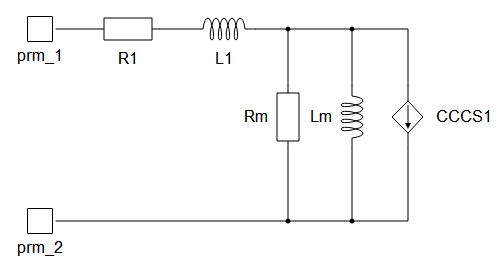Non-Linear / Hysteresis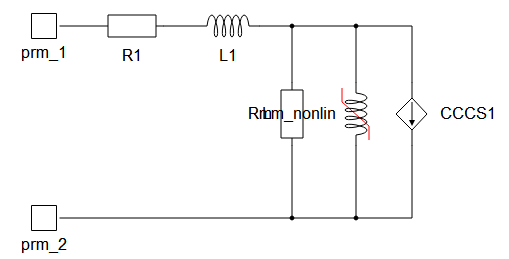Lm/Rm neglected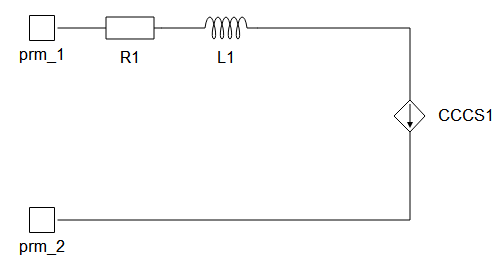## Hysteresis effects

Simulating hysteresis effects requires signal processing implementation. Therefore, an execution rate for hysteresis simulation must be specified. To capture this effect for an arbitrary dynamic signal, its period should be at least 20 times faster than the execution rate of hysteresis sampling, defined in Execution rate. Hysteresis is further defined through three lists/arrays which have different labels depending on the type of transformer or nonlinear inductor as shown in Figure 1.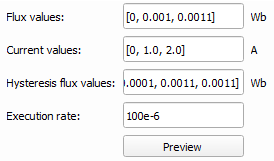Figure 1. Properties regarding hysteresis effectsHysteresis flux values define the hysteresis upper curve for the positive current, positive flux quadrant. The hysteresis bottom curve and the three other flux-current quadrants are extrapolated using symmetry. When hysteresis is enabled, the current source is created in parallel with a nonlinear inductor, injecting current only due to hysteresis effects with an execution rate specified through the Execution rate parameter. Nonlinear current functionality, however, will always be simulated with the solver execution rate, making saturation effects significantly more responsive than the hysteresis effect.

To properly define hysteresis, the data value for both Flux values and Hysteresis flux values must be specified for the zero current data-point, which means that the first value of Current values and Flux values lists/arrays must be 0, and that the first value of the Hysteresis flux values list/array must be the remanent flux value. Secondly, all three of these properties must have the same number of elements and all three must be monotonically non-decreasing lists/arrays. Hysteresis flux values must have at least one element that is higher in value than the corresponding element in the Flux values list, followed by at least one element that has the same value as the corresponding element in Flux values. The first common element for Hysteresis flux values and Flux values is defined as the Hysteresis upper curve threshold.

Figure 2 demonstrates an example of properly defined parameter values using the settings below.

Flux values [Wb]: [0, 0.004, 0.008, 0.011, 0.013, 0.014, 0.0145, 0.015]
Current values [A]: [0, 1, 2, 3, 4, 5, 6, 7]
Hysteresis flux values [Wb]: [0.004, 0.007, 0.01, 0.012, 0.0135, 0.0141, 0.0145, 0.015]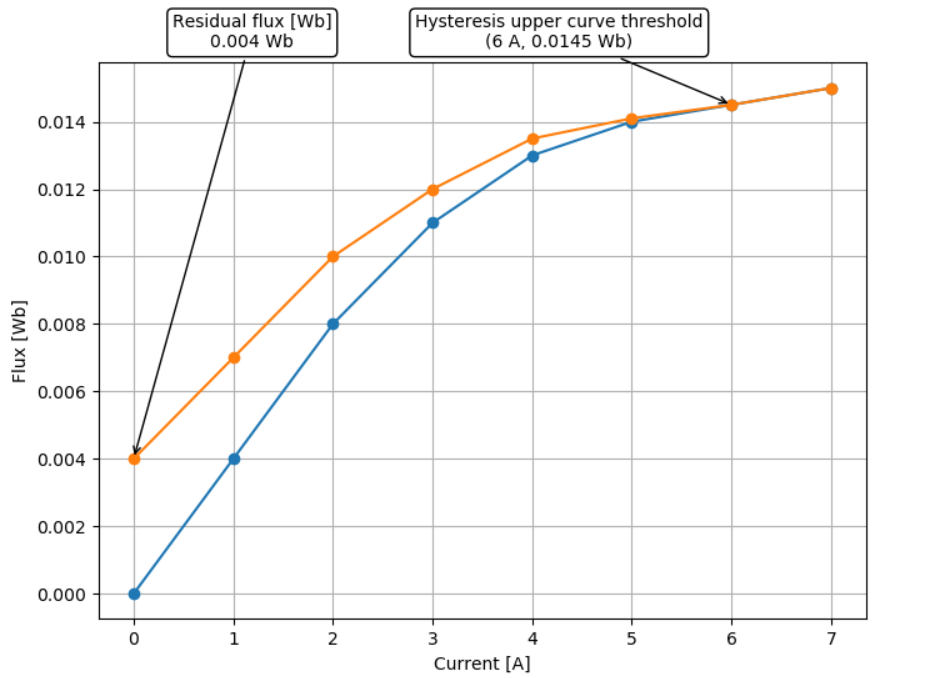Figure 2. Graphical example of properly defined parameter valuesClicking the Preview button will validate the hysteresis parameters, but only if they are defined directly in the respective property fields and not if they are defined through the namespace. After validation, the Hysteresis curve will be extended, showing the expected simulation hysteresis curve as shown in Figure 3.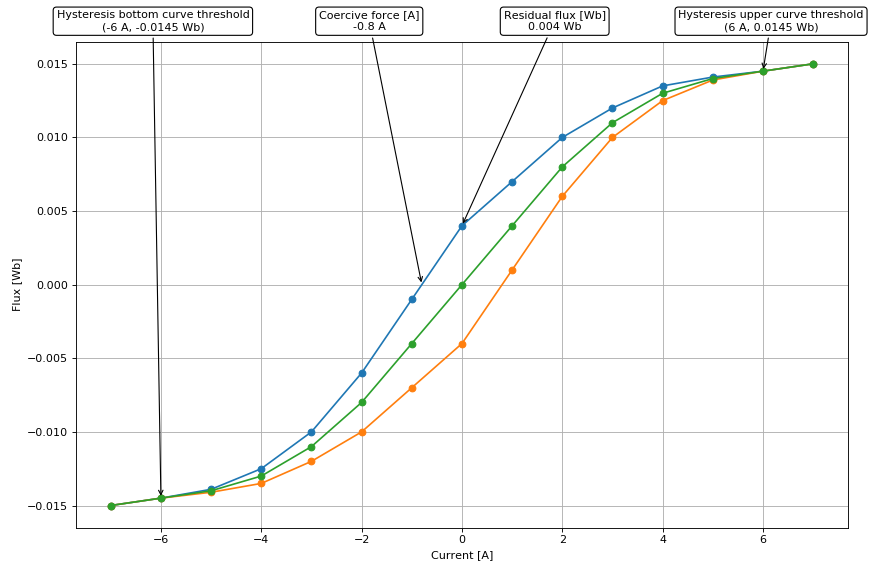Figure 3. Full flux current curve with hysteresis previewWhen the simulated flux value surpasses the Hysteresis upper curve threshold, the upper curve becomes the trajectory for the flux-current set-points and stays that way until the next trigger event. When the simulated flux value falls bellow the Hysteresis bottom curve threshold, the bottom curve becomes the trajectory for the flux-current set-points and stays that way until the next trigger event. When the simulation is first started, the original curve is a reference, until the next trigger event. A way to disable hysteresis and return the reference curve to the original magnetization curve during runtime, is to set the scada input named demagnetize_scada_in to 1. To re-enable the hysteresis upper and bottom curve, set the scada input demagnetize_scada_in to 0.

The current version of Schematic Editor offers a selection of the following types of transformer components:

Ideal transformer

Ideal transformer with variable transfer ratio

Phase-shifting Transformer

Single phase two winding transformer

Single phase two winding variable ratio transformer

Single phase three winding transformer

Single phase four winding transformer

Three phase two winding transformer

Three phase three winding transformer

Three phase four winding transformer

Single phase multi-winding transformer

Three phase two winding variable ratio transformer

Additional information on calculation of equivalent circuit parameter values is contained in a separate section.